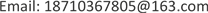1. 引言

2. 基本算法2.1. 自动子空间划分(Automatic Subspace Partition, ASP)

r i , j = ∑ k = 1 r × c ( b i k − b ¯ i ) ( b j k − b ¯ j ) ∑ k = 1 r × c ( b i k − b ¯ i ) 2 ∑ k = 1 r × c ( b j k − b ¯ j ) 2 (1)

R = ( r 11 … r 1 n ⋮ ⋱ ⋮ r n 1 ⋯ r n n ) (2)

R a d j ( i ) = f i , j + 1 = ∑ j = 1 r × c ( b j i − b i ¯ ) ( b j i + 1 − b i + 1 ¯ ) ∑ j = 1 r × c ( b j i − b i ¯ ) 2 ∑ j = 1 r × c ( b j i + 1 − b i + 1 ¯ ) 2 (3)

2.2. 最佳指数法(Optimum Index Factor, OIF)

1982年，Chaces等提出了最佳指数法。该方法完全遵守波段选择的原则，平衡信息量和相关性，原理简单，容易理解。OIF算法如公式(4)所示：

O I F = ∑ i = 1 p I i ∑ i = 1 p ∑ j = i + 1 p | R i , j | (4)

I ( i ) = 1 r c ∑ x = 1 r ∑ y = 1 c ( f i ( x , y ) − f ¯ i ) 2 (5)

f ¯ i = 1 r c ∑ x = 1 r ∑ y = 1 c f i ( x , y ) (7)

2.3. 信息熵

H = − ∑ i = 1 n p ( i ) * log 10 p ( i ) (8)

∑ i = 1 n p ( i ) = 1 (9)

i代表某个事件，p(i)代表事件i出现的概率。其中，对数的底一般为2或10，在该论文中，我们以10为底。信息熵是为表示信息量的多少而产生的，相较于特征值更具有说服力，且信息熵已形成一个完整的体系，便于算法的进一步完善、改进。

2.4. 巴氏系数

ρ B = ∑ i = 1 n p ( i ) q ( i ) (10)

∑ i = 1 n q ( i ) = 1 (11)

∑ i = 1 n p ( i ) = 1 (12)

3. BSEF算法3.1. BSEF算法的提出

X i j = ∑ d = 1 m p i ( d ) * p j ( d ) (13)

O I F = ∑ i = 1 p H ( i ) / ∑ i = 1 p ∑ j = i + 1 p X i , j (14)

H(i)代表选取波段的信息熵，p代表选取波段的个数。在实际的数据降维中，一般选取3-5个波段，在本文中，我们选取3个波段。与OIF降维方法相比，对波段块中信息量最大的波段进行组合，大大降低了组合的可能性，从而降低了数据降维时间。算法流程图如图1所示。

3.2. 试验及结果分析

Selection results of different band selection method

OIF16, 17, 1811003.330.9995, 0.8798, 0.8870
PCA-0.1681-
BSEF146, 177, 2020.20070.9599, 0.9359, 0.9850

AUC值表示ROC曲线下的面积，AUC值越大，表示算法检测效率越高。表2中不同算法AUC值与图4检测结果、图5中ROC曲线一致；四种算法的检测效果由好到坏依次为：BSEF-RX算法、PCA-RX算法、RX算法、XinxiShang-RX算法和FangCha-RX算法。

Performance parameters of different algorithm

AUC值0.97890.97630.96710.86030.8157

4. BERF算法4.1. BERF算法的提出

BERF算法的步骤如下：

4.2. BERF降维实验

Performance value of different algorithm

AUC值0.9998435540.9998239980.9997848870.8788

5. 结论